#### One-dimensional decomposition

To explain the cylindrical algebraic decomposition method, we first perform a semi-algebraic decomposition of, which is the final step in the projection sequence. Once this is explained, then the multi-dimensional case follows more easily.

Let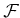be a set of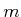univariate polynomials,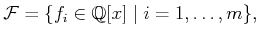(6.18)

which are used to define some semi-algebraic set in. The polynomials incould come directly from a quantifier-free formula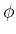(which could even appear inside of a Tarski sentence, as in (6.9)).

Define a single polynomial as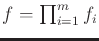. Suppose that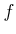hasdistinct, real roots, which are sorted in increasing order: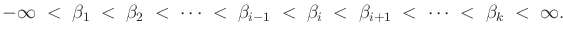(6.19)

The one-dimensional semi-algebraic decomposition is given by the following sequence of alternating-cells and 0-cells: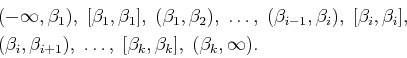(6.20)

Any semi-algebraic set that can be expressed using the polynomials incan also be expressed as the union of some of the 0-cells and-cells given in (6.20). This can also be considered as a singular complex (it can even be considered as a simplicial complex, but this does not extend to higher dimensions).

Sample points can be generated for each of the cells as follows. For the unbounded cells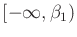and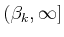, valid samples are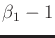and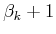, respectively. For each finite-cell,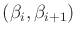, the midpointproduces a sample point. For each 0-cell,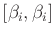, the only choice is to use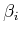as the sample point.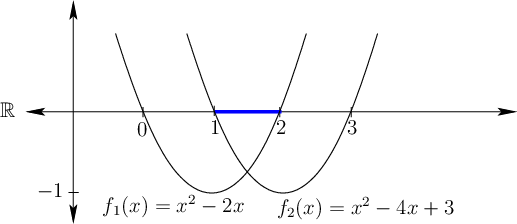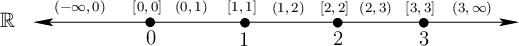Example 6..5 (One-Dimensional Decomposition)   Figure 6.31 shows a semi-algebraic subset ofthat is defined by two polynomials,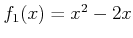and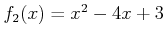. Here,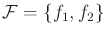. Consider the quantifier-free formula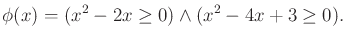(6.21)

The semi-algebraic decomposition into five-cells and four 0-cells is shown in Figure 6.32. Each cell is sign invariant. The sample points for the-cells are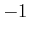,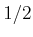,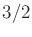,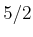, and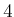, respectively. The sample points for the 0-cells are 0,,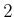, and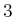, respectively.

A decision problem can be nicely solved using the decomposition. Suppose a Tarski sentence that uses the polynomials inhas been given. Here is one possibility: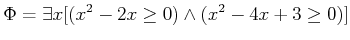(6.22)

The sample points alone are sufficient to determine whether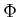is TRUE or FALSE. Once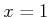is attempted, it is discovered thatis TRUE. The quantifier-elimination problem cannot yet be considered because more dimensions are needed.Steven M LaValle 2020-08-14